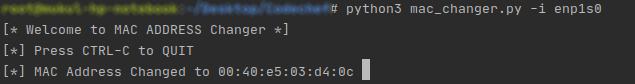Open in App
Not now

# Python script to change MAC address of Linux machine

• Difficulty Level : Expert
• Last Updated : 27 Jul, 2020

What is MAC Address: In a computer network, the MAC Address is used at the lowest networking level, where network interfaces communicate with one another. See details here.

• To bypass MAC Address filtering
• To bypass certain kind of MITM spoofing attack
• To avoid device tracking in a public network

There are many other tasks like becoming anonymous in a network and to avoid some network attacks where changing MAC Address becomes useful.

Changing MAC Address in Linux Machine: In Linux, a simple way to change MAC address is by using `ifconfig` command.
If it is not already installed, we can install it using:

```sudo apt-get update
sudo apt-get install ifconfig
```

After installing this package, we can change the MAC Address using:

```sudo ifconfig <interface-name> down
sudo ifconfig <interface-name> hw ether <new-mac-address>
sudo ifconfig <interface-name> up
```

We can see the list of interfaces using:

`ifconfig`

Automation using Python: Since it is not possible for us to manually change the MAC Address every time, we can automate the process of changing the MAC Address using Python. This script will keep changing the MAC Address in a constant period of time.
Below is the implementation of above idea.

 `# Python Script to change MAC Address`` ` `# Import Useful modules``import` `sys``import` `subprocess``import` `argparse``import` `random``import` `time``import` `re`` ` ` ` `# Function to get the interface name``def` `get_arguments():``    ``# This will give user a neat CLI``    ``parser ``=` `argparse.ArgumentParser()``    ``# We need the interface name``    ``parser.add_argument(``"-i"``, ``"--interface"``,``                ``dest``=``"interface"``,``                ``help``=``"Name of the interface. "``                ``"Type ifconfig for more details."``)``    ``options ``=` `parser.parse_args()``    ``# Check if interface was given``    ``if` `options.interface:``        ``return` `options.interface``    ``else``:``        ``parser.error(``"[!] Invalid Syntax. "``                     ``"Use --help for more details."``)`` ` ` ` `# Function to change the MAC Address``def` `change_mac(interface, new_mac_address):``    ``# As explained above, these lines will``    ``# execute these commands for us``    ``subprocess.call([``"sudo"``, ``"ifconfig"``, interface,``                     ``"down"``])``    ``subprocess.call([``"sudo"``, ``"ifconfig"``, interface,``                     ``"hw"``, ``"ether"``, new_mac_address])``    ``subprocess.call([``"sudo"``, ``"ifconfig"``, interface,``                     ``"up"``])`` ` `# Function to generate a random MAC Address``def` `get_random_mac_address():``    ``characters ``=` `"0123456789abcdef"``    ``random_mac_address ``=` `"00"``    ``for` `i ``in` `range``(``5``):``        ``random_mac_address ``+``=` `":"` `+` `\``                        ``random.choice(characters) \``                        ``+` `random.choice(characters)``    ``return` `random_mac_address`` ` `# Function to get the current MAC Address``# We will use it restore MAC address``# in case something goes wrong.``def` `get_current_mac(interface):``    ``output ``=` `subprocess.check_output([``"ifconfig"``,``                                      ``interface])``    ``return` `re.search(``"\w\w:\w\w:\w\w:\w\w:\w\w:\w\w"``,``                  ``str``(output)).group(``0``)`` ` `# Driver Program``if` `__name__ ``=``=` `"__main__"``:``    ``print``(``"[* Welcome to MAC ADDRESS Changer *]"``)``    ``print``(``"[*] Press CTRL-C to QUIT"``)``    ``# Change it to required value(in sec)``    ``TIME_TO_WAIT ``=` `60``    ``interface ``=` `get_arguments()``    ``current_mac ``=` `get_current_mac(interface)``    ``try``:``        ``while` `True``:``            ``random_mac ``=` `get_random_mac_address()``            ``change_mac(interface, random_mac)``            ``new_mac_summary ``=` `subprocess.check_output(``                ``[``"ifconfig"``, interface])``            ``if` `random_mac ``in` `str``(new_mac_summary):``                ``print``(``"\r[*] MAC Address Changed to"``,``                      ``random_mac,``                      ``end``=``" "``)``                ``sys.stdout.flush()``            ``# Wait for a constant period of time``            ``time.sleep(TIME_TO_WAIT)`` ` `    ``except` `KeyboardInterrupt:``        ``# Restore the MAC before quitting.``        ``change_mac(interface, current_mac)``        ``print``(``"\n[+] Quitting Program..."``)`

Output:My Personal Notes arrow_drop_up